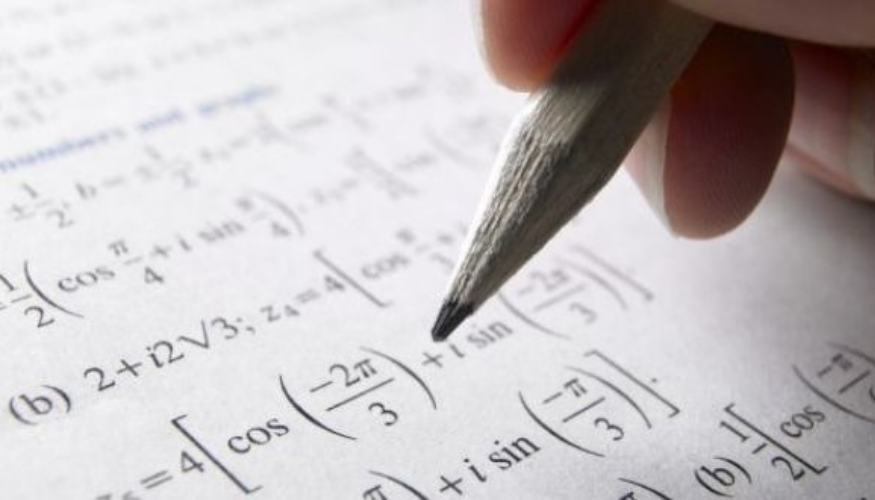#### Related Top Articles• 0
• 358

# Hello world### What is Trigonometry?

Trigonometry is a branch of mathematics that studies relationships between side lengths and angles of triangles.

### How Trigonometry is useful?

Primarily, trigonometry is a scientific study used to measure bearings and precise distances. Trigonometry uses the three basic functions of Sine, Cosine, and Tangent to make its calculations

### How do you calculate height using Trignometry?

When you see a tall object, such as a tree or a flagpole, you may wonder how tall the object is but not have any way to reach the top to measure the height. Instead, you can use trigonometry to calculate the height of the object.

The tangent function abbreviated "tan" on most calculators, is the ratio between the opposite and adjacent sides of a right triangle. If you know, or can measure the distance from the object to where you are, you can calculate the height of the object.

Measure the distance from the object you want to calculate the height of where you are standing.

Use the protractor to estimate the angle formed by the line parallel to the ground at your eye level and the line from the top of the object to your eyes.

Use your calculator to find the tangent of the angle from step two. For example, if the angle from step two was 35 degrees, you would get approximately 0.700.

Multiply your distance from the object by the result from step three.

For example, if you were 20 feet from the object, you would multiply 20 by 0.700 to get about 14 feet.

Measure the distance from the ground to your eyeball and add the result to the result from step four to calculate the height of the object.

For example, if you measure five feet from the ground to your eyeballs, you would add five to 14 to find the total height of the object equals to 19 feet.

### Fields That Use Trigonometry:

Scientific fields that make use of trigonometry include:

acoustics, architecture, astronomy, cartography, civil engineering, geophysics, crystallography, electrical engineering, electronics, land surveying and geodesy, many physical sciences, mechanical engineering, machining, medical imaging, number theory, oceanography, optics, pharmacology, probability theory, seismology, statistics, and visual perception

### Career In Trigonometry:

Trigonometry is used by engineers, medical services technicians, mathematicians, data entry specialists, loggers, statisticians, actuaries, drafters, chemists, economists, physicists, registered nurses, building inspectors, boilermakers, machinists and millwrights.